# Hahn-Banach theorem

(diff) ← Older revision | Latest revision (diff) | Newer revision → (diff)

Letbe a linear manifold in a real or complex vector space. Supposeis a semi-norm onand supposeis a linear functional defined onwhich satisfies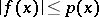(*)

for every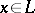. Thencan be extended to a linear functionalon all ofsuch thatfor all. Such is an extension is not uniquely determined.

In the case of a real spacethe semi-norm can be replaced by a positively-homogeneous subadditive function, and the inequality (*) by the one-sided inequality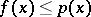, which remains valid for the extended functional. Ifis a Banach space, then for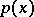one can take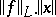, and then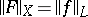. The theorem was proved by H. Hahn (1927), and independently by S. Banach (1929).

How to Cite This Entry:
Hahn-Banach theorem. Encyclopedia of Mathematics. URL: http://encyclopediaofmath.org/index.php?title=Hahn-Banach_theorem&oldid=15516
This article was adapted from an original article by V.I. Sobolev (originator), which appeared in Encyclopedia of Mathematics - ISBN 1402006098. See original article